# NegativeBinomialTest

Unpaired hypothesis test result

## Description

A `NegativeBinomialTest` object, returned by the `nbintest` function, contains the results of an unpaired hypothesis test for short-read count data with small sample sizes. Use this object to access p-values of the test or to create diagnostic plots.

## Creation

`nbintest` returns the unpaired hypothesis test result as a `NegativeBinomialTest` object. You cannot construct this object directly.

## Properties

expand all

Two-sided p-values, specified as a column vector, for every row of the inputs to `nbintest`.

Linkage type between the variance and mean, specified as a character vector or string. This table summarizes the available linkage options.

`'LocalRegression'`The variance is the sum of the shot noise term (mean) and a locally regressed nonparametric smooth function of the mean as described in . This option is the default. Use this option if your data contains several rows (genes), such as more than 1000 rows.
`'Constant'`The variance is the sum of the shot noise term (mean) and a constant multiplied by the squared mean as described in . This method uses all the rows in the data to estimate the constant. Use this option if your data has fewer rows, that is, less than 1000 rows, and is overdispersed.
`'Identity'`The variance is equal to the mean as described in . Counts are therefore modeled by the Poisson distribution individually for each row of `X` and `Y`. Use this option to compare the results of the other two options.

Logical flag to pool variance between both conditions, specified as 1 (`true`) or 0 (`false`). The default is 0, meaning the variance is estimated separately for each condition.

Size (scaling) factor of each column in `X` and `Y`, specified as a cell array of two vectors, such as `{SX,SY}`. `SX` and `SY` are numeric vectors with sizes equal to `size(X,2)` and `size(Y,2)`.

Note

These properties are read-only. Run `nbintest` to change them.

## Object Functions

 `plotVarianceLink` Plot the sample variance versus the estimate of the condition-dependent mean `plotChiSquaredFit` Plot goodness-of-fit for variance regression

## Examples

collapse all

This example shows how to perform an unpaired hypothesis test for synthetic short-read count data from two different biological conditions.

The data in this example contains synthetic gene count data for 5000 genes, representing two different biological conditions, such as diseased and normal cells. For each condition, there are five samples. Only 10% of the genes (500 genes) are differentially expressed. Specifically, half of them (250 genes) are exactly 3-fold overexpressed. The other 250 genes are 3-fold underexpressed. The rest of the gene expression data is generated from the same negative binomial distribution for both conditions. Each sample also has a different size factor (that is, the coverage or sampling depth).

`load('nbintest_data.mat','K','H0');`

The variable `K` contains gene count data. The rows represent genes, and the columns represent samples. In this case, the first five columns represent samples from the first condition. The other five columns represent samples from the second condition. Display the first few rows of `K`.

`K(1:5,:)`
```ans = 5×10 13683 14140 8281 14309 12208 8045 9446 11317 14597 14592 16028 16805 9813 16486 14076 9901 10927 13348 16999 17036 814 862 492 910 758 521 573 753 870 936 15870 16453 9857 16454 14267 9671 10997 13624 17151 17205 9422 9393 5734 9598 8174 5381 6315 7752 9869 9795 ```

In this example, the null hypothesis is true when the gene is not differentially expressed. The variable `H0` contains boolean indicators that indicate for which genes the null hypothesis is true (marked as 1). In other words, H0 contains known labels that you will use later to compare with predicted results.

`sum(H0)`
```ans = 4500 ```

Out of 5000 genes, 4500 are not differentially expressed in this synthetic data.

Run an unpaired hypothesis test for samples from two conditions using `nbintest`. The assumption is that the data came from a negative binomial distribution, where the variance is linked to the mean via a locally-regressed smooth function of the mean as described in  by setting `'VarianceLink'` to `'LocalRegression'`.

`tLocal = nbintest(K(:,1:5),K(:,6:10),'VarianceLink','LocalRegression');`

Use `plotVarianceLink` to plot a scatter plot for each experimental condition (for X and Y conditions), with the sample variance on the common scale versus the estimate of the condition-dependent mean. Use a linear scale for both axes. Include curves for all other linkage options by setting `'Compare'` to `true`.

`plotVarianceLink(tLocal,'Scale','linear','Compare',true)`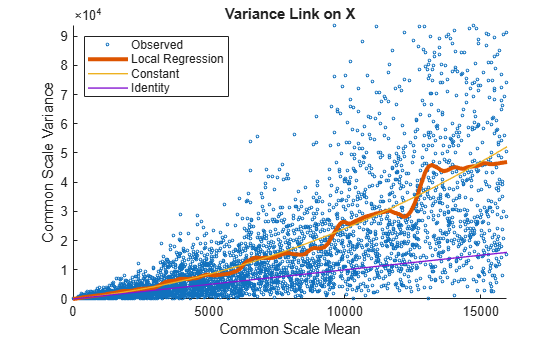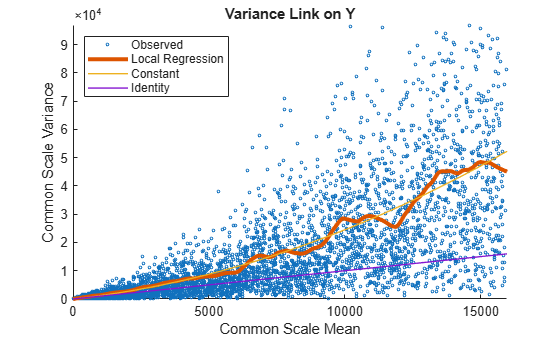The `Identity` line represents the Poisson model, where the variance is identical to the mean as described in . Observe that the data seems to be overdispersed (that is, most points are above the `Identity` line). The `Constant` line represents the negative binomial model, where the variance is the sum of the shot noise term (mean) and a constant multiplied by the squared mean as described in . The `Local Regression` and `Constant` linkage options appear to fit better with the overdispersed data.

Use `plotChiSquaredFit` to assess the goodness-of-fit for variance regression. It plots the empirical CDF (ecdf) of the chi-squared probabilities. The probabilities are the ratio between the observed and the estimated variance stratified by short-read count levels into five equal-sized bins.

`plotChiSquaredFit(tLocal)`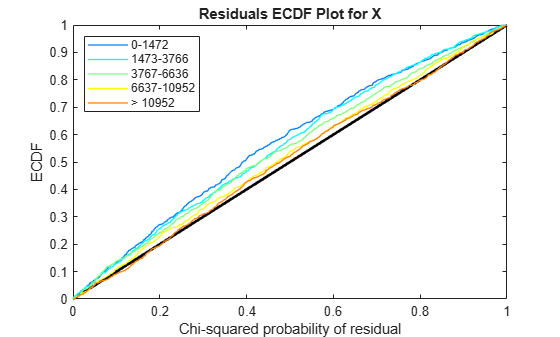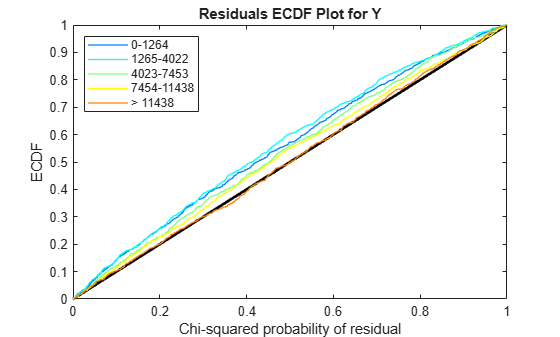Each figure shows five ecdf curves. Each curve represents one of the five short-read count levels. For instance, the blue line represents the ecdf curve for a low short-read counts between 0 and 1264. The red line represents high counts (more than 11438).

One way to interpret the curves is to check if the ecdf curves are above the diagonal line. If they are above the line, then the variance is overestimated. If they are below the line, then the variance is underestimated. In both figures, the variance seems to be correctly estimated for higher counts (that is, the red line follows the diagonal line), but slightly overestimated for lower count levels.

To assess the performance of the hypothesis test, construct a confusion matrix using the known labels and the predicted p-values.

`confusionmat(H0,(tLocal.pValue > .001))`
```ans = 2×2 493 7 5 4495 ```

Out of 500 differentially expressed genes, 493 are correctly predicted (true positives) and 7 of them are incorrectly predicted as not-differentially expressed genes (false negatives). Out of 4500 genes that are not differentially expressed, 4495 are correctly predicted (true negatives) and 5 of them are incorrectly predicted as differentially expressed genes (false positives).

For a comparison, run the hypothesis test again assuming that counts are modeled by the Poisson distribution, where the variance is identical to the mean.

`tPoisson = nbintest(K(:,1:5),K(:,6:10),'VarianceLink','Identity');`

Plot the ecdf curves. Observe that all the curves are below the diagonal line, implying that the variance is underestimated. Therefore, the negative binomial model fits the data better.

`plotChiSquaredFit(tPoisson)`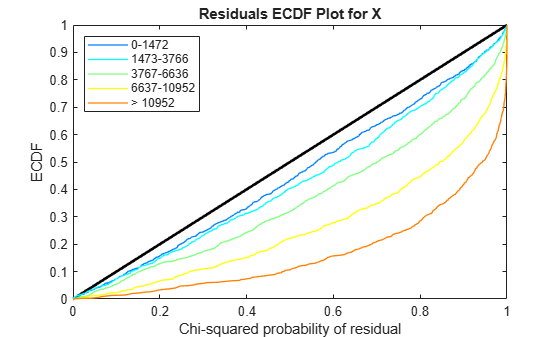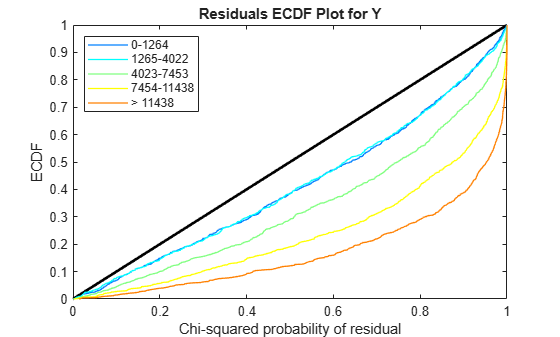Anders, S., and Huber, W. (2010). Differential Expression Analysis for Sequence Count Data. Genome Biology, 11(10):R106.

 Robinson, M.D., and Smyth, G.K. (2008). Small-sample Estimation of Negative Binomial Dispersion, with Applications to SAGE data. Biostatistics, 9:321-332.

 Marioni, J.C., Mason, C.E., Mane, S.M., Stephens, M., and Gilad, Y. (2008). RNA-seq: an Assessment of Technical Reproducibility and Comparison with Gene Expression Arrays. Genome Research, 16:1509-1517.